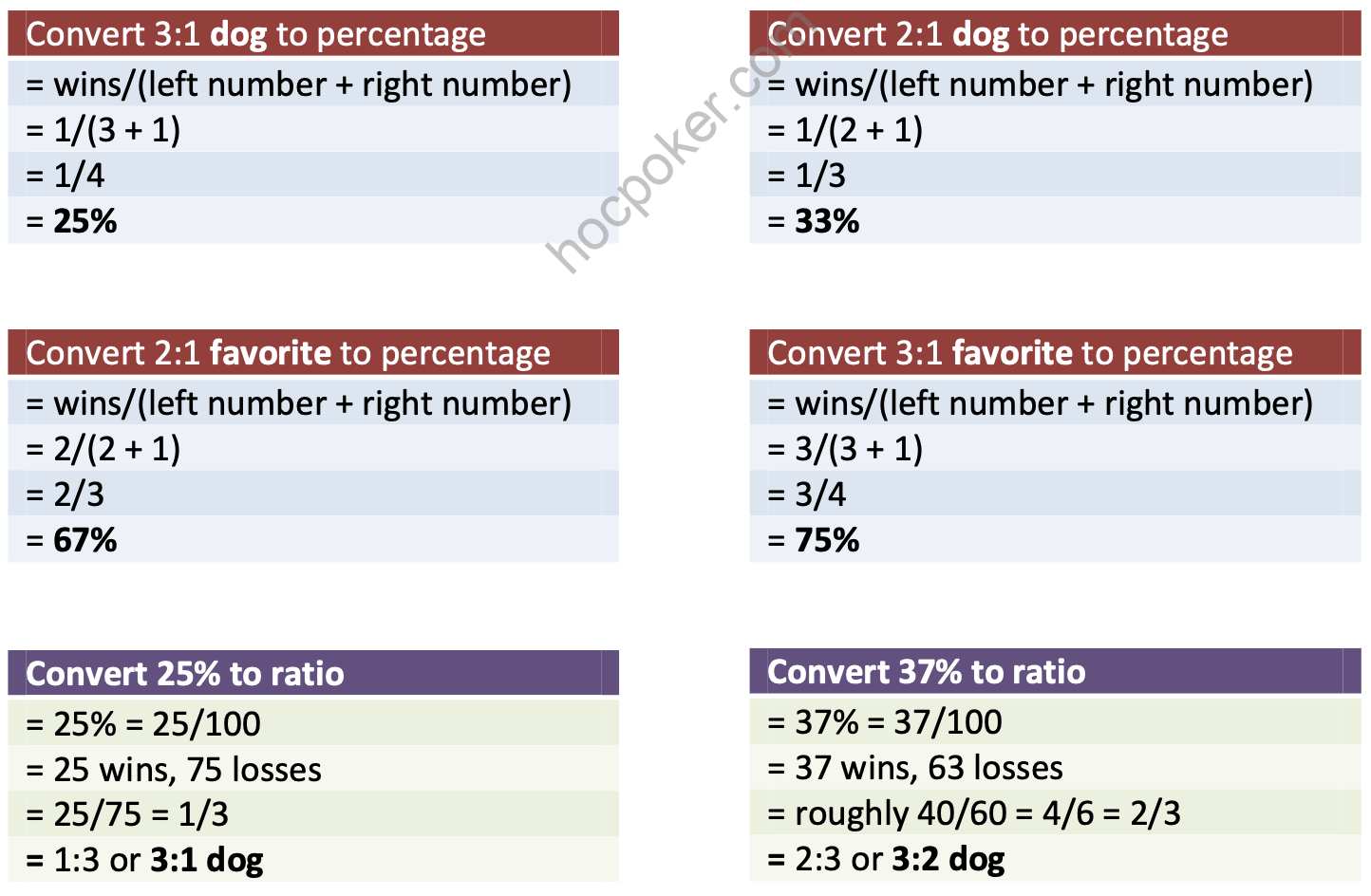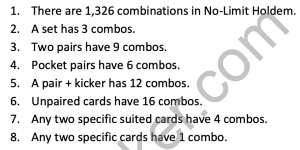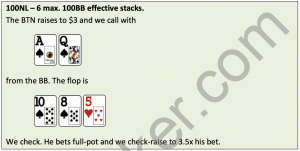# Math is Easy – The FundamentalsIf you’re not good with numbers, don’t worry. You only need to understand a few basic fundamentals of poker to become a great player. Once you learn these concepts, you will no longer have to worry about the mathematical aspects of poker.

Probability and Odds

Probability is the proportion of the time that something is likely to occur or has already occurred. It is usually expressed as a percentage (e.g., 40 percent) or a ratio indicating odds (e.g., 7:1). They are one and the same. For example, an open-ended straight draw has 31.5 percent equity on the flop, which is equivalent to saying it’s a 2.2:1 dog. Percentages are very straightforward. The higher the percentage, the more often an event is likely to occur. Now let’s go over ratios.

There are two ways to describe a ratio. You can say it in terms of being a favorite or a dog, and there’s a significant difference between the two. The simplest thing to keep in mind is that when you’re a favorite, you’re ahead, and when you’re a dog, you’re behind.

Let’s look at a few examples.

• To be a 2:1 favorite means that for every two times that you win, you lose once.
• To be a 3:1 dog implies that you lose three times for every one time that you win. Now, let’s say we want to convert a 4:1 dog to a percentage. To do that, we use the equation below:

Percentage = wins/(left number + right number)

Convert 4:1 dog to a percentage.

percentage = wins/(left number + right number)

= 1/(4 + 1)

= 1/5

= 20%

Let’s have some more practice.

Pot Odds

One of the essentials of poker, pot odds are something that every poker player should learn about. Whether you’re grinding it at a 10NL table or playing high-stakes, you have to know what pot odds are. We will cover two types of odds: immediate odds and implied odds. Knowing about odds allows us to determine if a call, bet, fold or raise is the most optimal play.

Immediate odds

Normally referred to as simply “odds”, immediate odds are how much you stand to win immediately in relation to what you have to risk, and is usually expressed as a ratio. Here are five simple steps we need to take to determine our pot odds.

Let’s apply this in a few examples.

Say we get to the river with top pair and there’s \$50 in a pot. Villain bets \$25. Do we have enough odds to call?

In order to know if you can profitably call here, you need to know your odds. To determine this, let’s follow the steps above.

1. Original size of pot = \$50
2. Amount we have to call = \$25
3. Original size of pot + amount we have to call = Total Pot Size \$50 + \$25 = \$75
4. Total Pot Size:Amount we have to call 75:25, or 3:1 odds.
5. 3:1 odds converts to 25 percent equity, which means if you are good here 25 percent of the time, you break even. If more than 25 percent, you stand to win money in the long run. With our top pair, we think we’re good here more than 25 percent of the time, so we call.

Sometimes the numbers don’t round up perfectly, so we’ll have to do some adjusting. Here’s an example:

We get to the river with top pair and the pot is \$70. Villain goes all-in for \$65. We think we’re good here about 50 percent of the time. Do we have enough odds to call?

We need to call \$65 to win \$135 (\$70 + \$65). That’s 135:65. This can be a hard number to work with, so let’s round it up. \$135 is about twice as big as \$65, so we reduce that to about 2:1. We can convert 2:1 odds to about 33 percent equity. Since we think we’re good here at least 50 percent of the time, we can profitably call.

Hopefully, that wasn’t too hard. It does take a little getting used to in the beginning, but after a bit of practice, you can easily do this in seconds. If you have trouble understanding this, I suggest you read this section again to feel more comfortable. It took me a while getting used to it myself.

Let’s go over another example for some more practice.

Let’s apply the steps from above:

1. Original size of pot = \$20
2. Amount we have to call = \$15
3. Original size of pot + amount we have to call = Total Pot Size \$20 + \$15 = \$35
4. Total Pot Size:Amount we have to call 35:15, or 2.33:1 odds. To get this number, just compute 35/15 on a calculator.
5. 2.33:1 odds converts to 30 percent equity, which means if we are good here 30 percent of the time, we break even. If more than 30 percent, we stand to win money in the long run. Against a hand like AQ, we have about 37 percent chance of winning, but ONLY if we get to see BOTH the turn and river. If we plan to fold to a turn bet when we miss, then we only get to see one card. This reduces our 37 percent equity to about 20 percent. Based on this alone, we can’t call. Good thing we have implied odds.

Implied Odds

Implied odds reflect how much we expect to win in later streets if we hit our hand. In the above example, if we know that Villain is the type of player who will stack off with Ax even when the flush card comes, we can call here all day. We have a lot of money left behind to make up for it. Although our immediate odds aren’t great, our implied odds are enough to at least warrant a call.

Figuring out implied odds is very similar to determining immediate odds. You simply add what he has left (excluding his bet) to the Total Pot Size.

So after he bets the flop, he still has \$75 left. So \$75 + \$35 (Total Pot Size) = \$110. Our new odds are 110:15, which is about 7.33-to-1. Since we only need *4:1 odds to call, we can continue.

* A flush draw has 19 percent equity if you get to see only 1 card; this is the same as a 4:1 odds. Check out The Odds Chart on page 33.

Reverse Implied Odds

Reverse implied odds reflect how much you stand to lose even if you do hit your hand. The most basic example is raising with ATo from UTG. We don’t want to do this in an aggressive game because this hand has a lot of reverse implied odds. Even if we do hit an Ace on the flop, we will stand to lose a lot of money against AJ, AQ and AK when the money goes in. Furthermore, we will not be able to extract much from weaker aces that will only call one or two small bets.

Here is a simple reverse implied odds situation that isn’t so obvious at first.

You should check-fold. Because there are so many players in the pot, your hand is rarely ahead. If you are behind, you are almost drawing dead. Thus, in this situation, you should check it down unless your hand improves on the turn or the river.Previous post Math is EasyNext post Math is Easy – Fold Equity